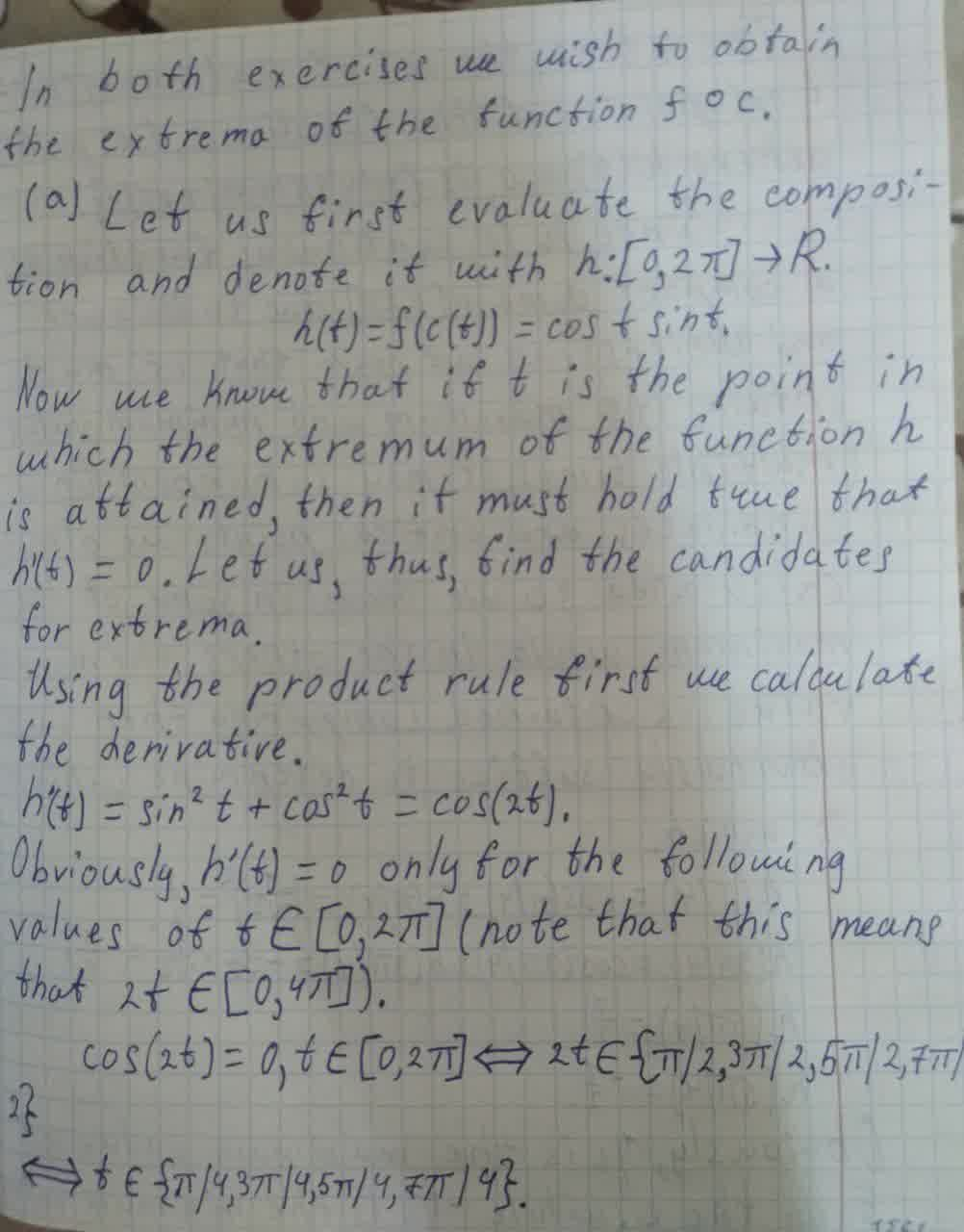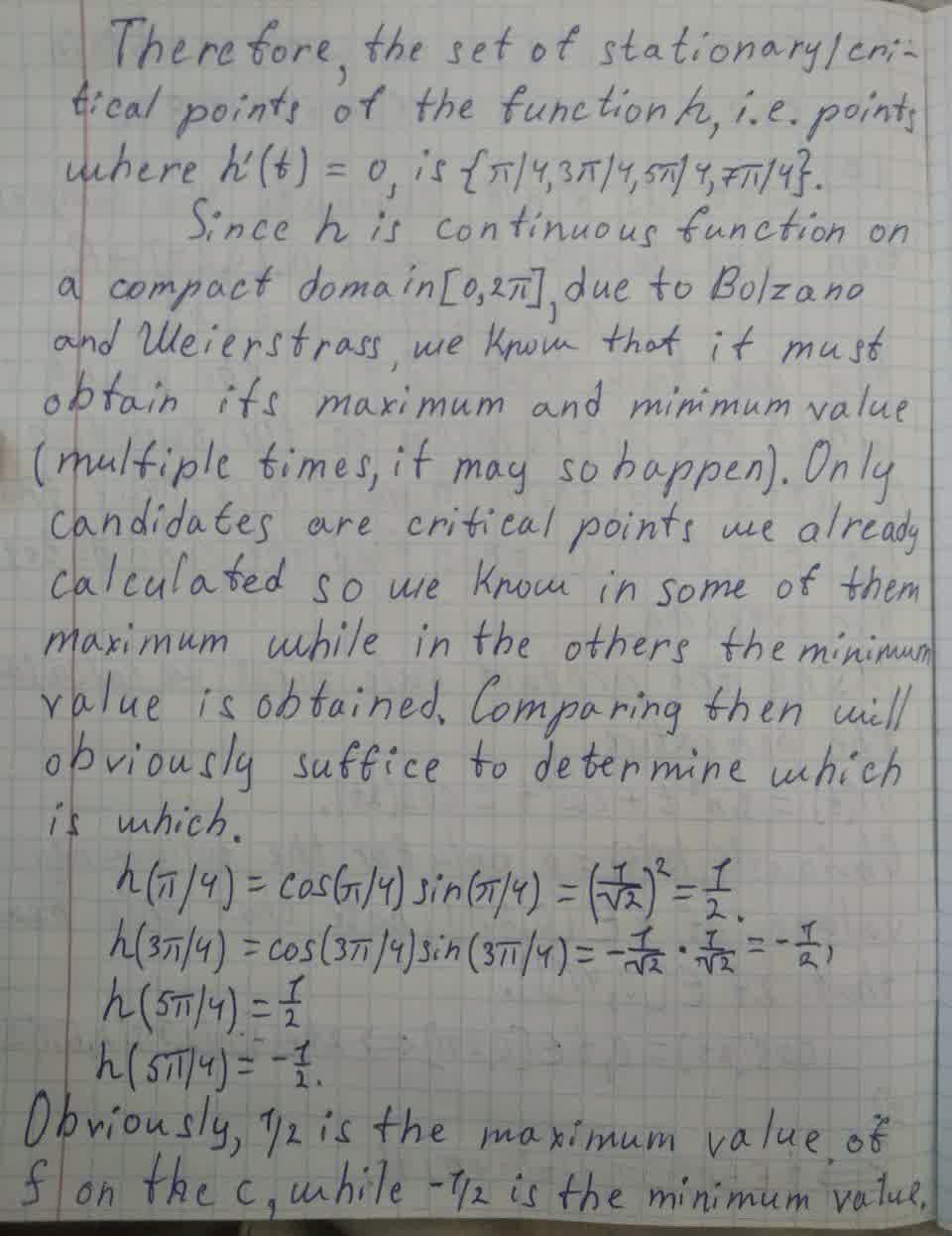Question# For each of the following, find the maximum and minimum values attained by the function f along the path c(t): (a)f(x,y) = xy. c(t) = (cost,sint). 0 \leq t \leq 2 \pi

Functions
ANSWEREDFor each of the following, find the maximum and minimum values attained by the function f along the path c(t):
(a) $$\displaystyle{f{{\left({x},{y}\right)}}}={x}{y}.{c}{\left({t}\right)}={\left({\cos{{t}}},{\sin{{t}}}\right)}.{0}\leq{t}\leq{2}\pi$$